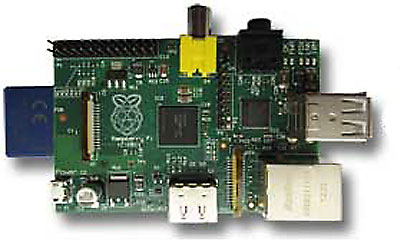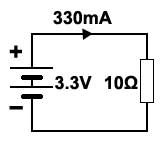# Conductors and Insulators

• Section 1.1 Conductors & Insulators.
• • Recognise common electrical conductors and their uses.
• • Recognise common electrical Insulators and their uses.
• Section 1.2 Materials in Circuits.
• • Identify electrical conductors on a PCB.
• • Identify common insulating and conducting materials and their uses on a PCB.
• Section 1.3 Resistance in Conductors.
• • Calculate the dimensions of a conductor.
• • Describe the effect of length and cross sectional area on the resistance of a conductor.
• Section 1.4 Resistivity.
• • Describe the property of resistivity.
• • Carry out calculations involving resistivity.
• • Use appropriate electrical units (Ωm) to describe the resistivity of common conductor & insulator materials
• Section 1.5 Temperature Effects
• • Describe the effect of temperature on the resistance of a conductor.
• • Describe the effect of temperature on the resistance of a insulator.
• • Define negative and positive temperature coefficients.
• Section 1.6 Resistivity Quiz
• • Calculations on Resistivity.## Introduction

Electronic circuits range from quite simple arrangements of a few connected components to vast and very complex networks. This module provides a basic introduction to circuits and their properties.## Simple Circuit

However even a complex circuit, such as the Raspberry Pi Shown in Fig. 1.0.1 can for some analysis purposes be illustrated by a simple diagram such as that shown in Fig. 1.0.2. This is because all of the complexity of a circuit can be replaced (in theory) by a single resistor.

The Raspberry Pi can be powered by a 3.3V DC supply, from which it draws around 330mA of current (depending on what mode it is operating in). This means that theoretically the Pi could be replaced by a resistor whose value would be:

3.3V divided by 330mA = 10 ohms.

Why? Admittedly the resistor would not do as much as the Raspberry Pi, but it will make circuit calculations much simpler!

Any electrical or electronic circuit, however complex, supplied with a driving voltage from some sort of power supply, will pass a certain amount of current, and that is the same action as if a power supply (mains/line, battery, radio signal or whatever) were supplying a single resistor having a particular value of resistance.

Using just the basic properties of simple circuits containing only power supplies, conductors, insulators and resistors can greatly simplify the understanding of more complex circuits. This initial module will therefore study the basic properties of conductors and insulators and show you how to calculate their important values. Later modules in this series will introduce resistors, both as single components and as part of more complex networks.